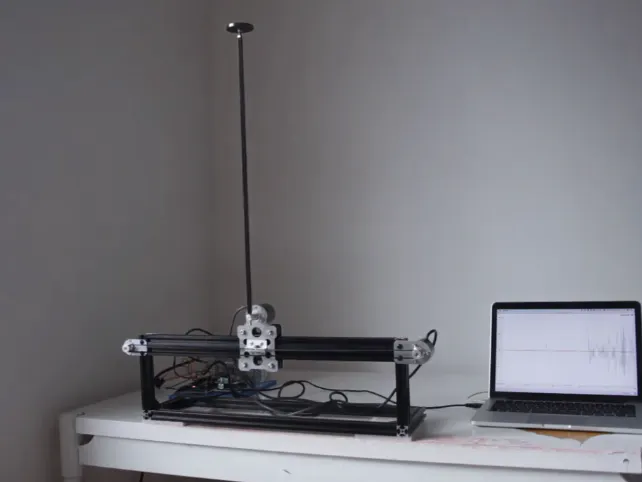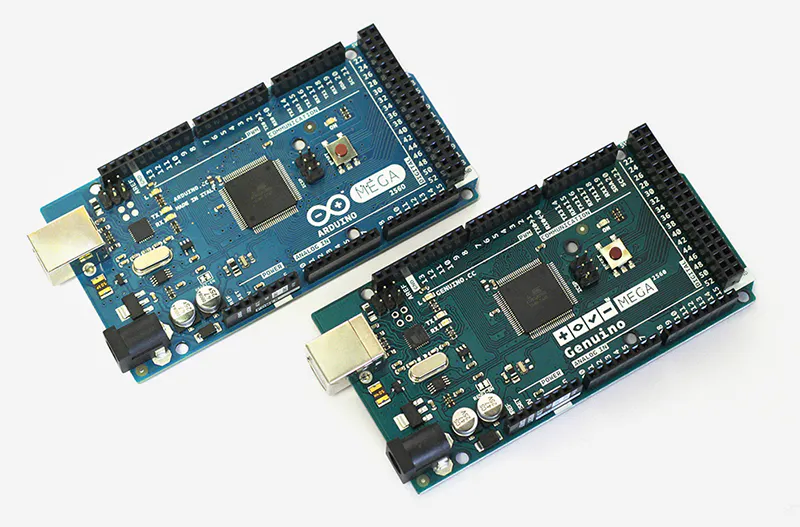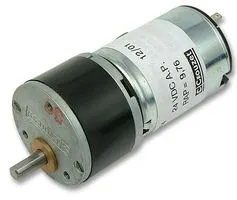# Inverted Pendulum on a Cart

Balancing an inverted pendulum on a cart with a DC motor. A perfect project for studying mechanical engineering and feedback control theory.

IntermediateShowcase (no instructions)6,166## Things used in this project

### Hardware componentsArduino Mega 2560 & Genuino Mega 2560
×1
 Omron Electronic Components LLC OMRON E6B2-CWZ6C
×1Geared DC Motor, 12 V
×1
 Cytron Technologies 10Amp 5V-30V DC Motor Driver
×1

### Software apps and online servicesArduino IDE

## Code

### Balance an inverted pendulum with a DC motor

Arduino
```/**
* == Inverted pendulum stabilisation with state control with DC motor ==
*
* == Hardware specification ==
* OMRON E6B2-CWZ6C pinout
* - Brown - Vcc
* - Black - Phase A
* - White - Phase B
* - Orange - Phaze Z
* - Blue - GND
*
* LPD3806-600BM-G5-24C pinout
* - Green - Phase A
* - White - Phase B
* - Red - Vcc
* - Black - GND
*/

#include <Arduino.h>

// motor encoder pins
#define OUTPUT_A  3 // PE5
#define OUTPUT_B  2 // PE4

// pendulum encoder pins
#define REF_OUT_A 18 // PD3
#define REF_OUT_B 19 // PD2

// pulses per revolution
#define PPR  2400
#define SHAFT_R 0.00573
#define PENDULUM_ENCODER_PPR  10000

#define PWM_PIN 10
#define DIR_PIN 8

#define POSITION_LIMIT  0.145

#define A 35.98
#define B 2.22
#define C 2.79

#define Kth 147.1
#define Kw  36.0
#define Kx  54.0
#define Kv  39.5

const float THETA_THRESHOLD = PI / 12;
const float PI2 = 2.0 * PI;

volatile long encoderValue = 0L;
volatile long lastEncoded = 0L;

volatile long refEncoderValue = 0;
volatile long lastRefEncoded = 0;

unsigned long now = 0L;
unsigned long lastTimeMicros = 0L;

float x, last_x, v, dt;
float theta, last_theta, w;
float control, u;

unsigned long log_prescaler = 0;

void encoderHandler();
void refEncoderHandler();

void setup() {

// setting PWD frequency on pin 10 to 31kHz
TCCR2B = (TCCR2B & 0b11111000) | 0x01;

pinMode(OUTPUT_A, INPUT_PULLUP);
pinMode(OUTPUT_B, INPUT_PULLUP);

pinMode(REF_OUT_A, INPUT_PULLUP);
pinMode(REF_OUT_B, INPUT_PULLUP);

attachInterrupt(digitalPinToInterrupt(OUTPUT_A), encoderHandler, CHANGE);
attachInterrupt(digitalPinToInterrupt(OUTPUT_B), encoderHandler, CHANGE);

attachInterrupt(digitalPinToInterrupt(REF_OUT_A), refEncoderHandler, CHANGE);
attachInterrupt(digitalPinToInterrupt(REF_OUT_B), refEncoderHandler, CHANGE);

pinMode(PWM_PIN, OUTPUT);
pinMode(DIR_PIN, OUTPUT);

digitalWrite(DIR_PIN, LOW);

Serial.begin(9600);
lastTimeMicros = 0L;
}

float saturate(float v, float maxValue) {
if (fabs(v) > maxValue) {
return (v > 0) ? maxValue : -maxValue;
} else {
return v;
}
}

float getAngle(long pulses, long ppr) {
float angle = (PI + PI2 * pulses / ppr);
while (angle > PI) {
angle -= PI2;
}
while (angle < -PI) {
angle += PI2;
}
return angle;
}

float getCartDistance(long pulses, long ppr) {
return 2.0 * PI * pulses / PPR * SHAFT_R;
}

void driveMotor(float u) {
digitalWrite(DIR_PIN, u > 0.0 ? LOW : HIGH);
analogWrite(PWM_PIN, fabs(u));
}

boolean isControllable(float theta) {
return fabs(theta) < THETA_THRESHOLD;
}

void log_state(float control, float u) {
if (fabs(w) > 100) {
return;
}

if (log_prescaler % 20 == 0) {
Serial.print(theta, 4);Serial.print("\t");
Serial.print(w, 4);Serial.print("\t");
Serial.print(x, 4);Serial.print("\t");
Serial.print(v, 4);Serial.print("\t");
Serial.print(control, 4);Serial.print("\t");
Serial.println(u, 4);
}
log_prescaler++;
}

void loop() {
now = micros();
dt = 1.0 * (now - lastTimeMicros) / 1000000;
x = getCartDistance(encoderValue, PPR);
v = (x - last_x) / dt;

theta = getAngle(refEncoderValue, PENDULUM_ENCODER_PPR);
w = (theta - last_theta) / dt;

if (isControllable(theta) && fabs(x) < POSITION_LIMIT) {
control = (Kx * x + Kv * v + Kth * theta + Kw * w);
u = (control + A * v + copysignf(C, v)) / B;
u = 255.0 * u / 12.0;
driveMotor(saturate(u, 254));
} else {
driveMotor(0);
}

last_x = x;
last_theta = theta;
lastTimeMicros = now;

log_state(control, u);

delay(5);
}

/**
* Motor encoder handler
*/
void encoderHandler() {
int MSB = (PINE & (1 << PE5)) >> PE5; //MSB = most significant bit
int LSB = (PINE & (1 << PE4)) >> PE4; //LSB = least significant bit
int encoded = (MSB << 1) | LSB; //converting the 2 pin value to single number
int sum  = (lastEncoded << 2) | encoded; //adding it to the previous encoded value

if(sum == 0b1101 || sum == 0b0100 || sum == 0b0010 || sum == 0b1011) {
encoderValue++; //CW
}
if(sum == 0b1110 || sum == 0b0111 || sum == 0b0001 || sum == 0b1000) {
encoderValue--; //CCW
}

lastEncoded = encoded; //store this value for next time
}

/**
* Pendulum encoder handler
* Encoder attached to pins:
* Phase A - 18 PD3
* Phase B - 19 PD2
*/
void refEncoderHandler() {
int MSB = (PIND & (1 << PD3)) >> PD3; //MSB = most significant bit
int LSB = (PIND & (1 << PD2)) >> PD2; //LSB = least significant bit
int encoded = (MSB << 1) | LSB; //converting the 2 pin value to single number
int sum  = (lastRefEncoded << 2) | encoded; //adding it to the previous encoded value

if(sum == 0b1101 || sum == 0b0100 || sum == 0b0010 || sum == 0b1011) {
refEncoderValue++; //CW
}
if(sum == 0b1110 || sum == 0b0111 || sum == 0b0001 || sum == 0b1000) {
refEncoderValue--; //CCW
}

lastRefEncoded = encoded; //store this value for next time
}
```

### Inverted pendulum repo

Contains all the sources and scripts used during the project

## Credits

### zjor

1 project • 8 followers